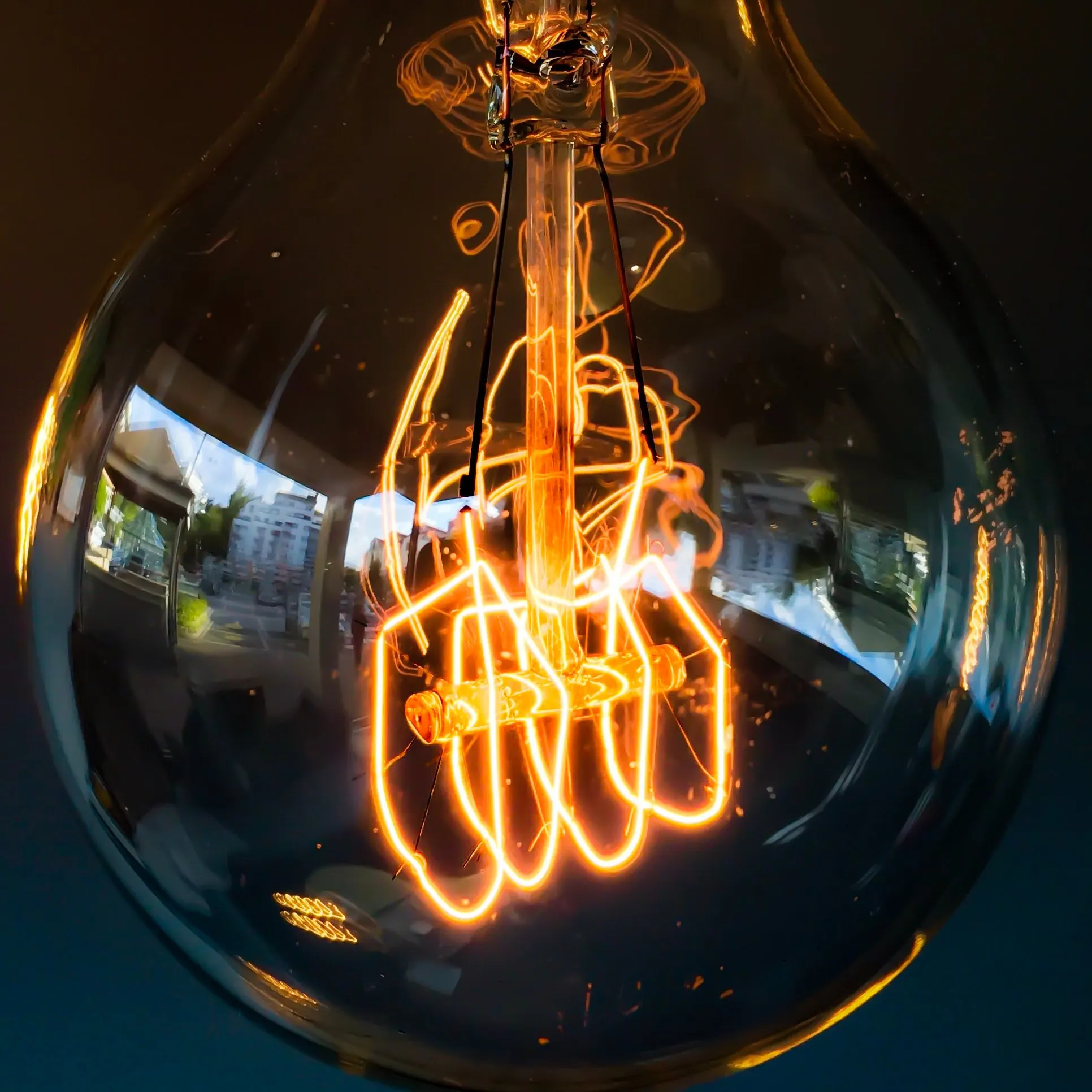Published © Apache-2.0

# Smart Trolley Using RFID And Arduino Nano

How to make Smart Trolley Using RFID And Arduino Nano

IntermediateFull instructions provided1 hour2,600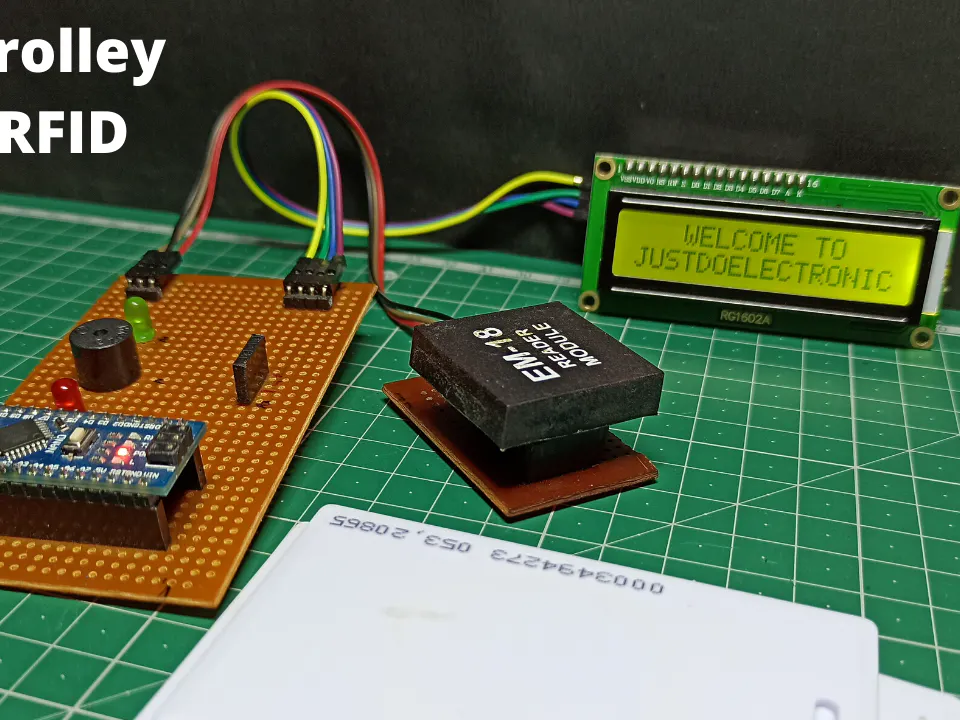## Things used in this project

### Hardware componentsArduino Nano R3
×1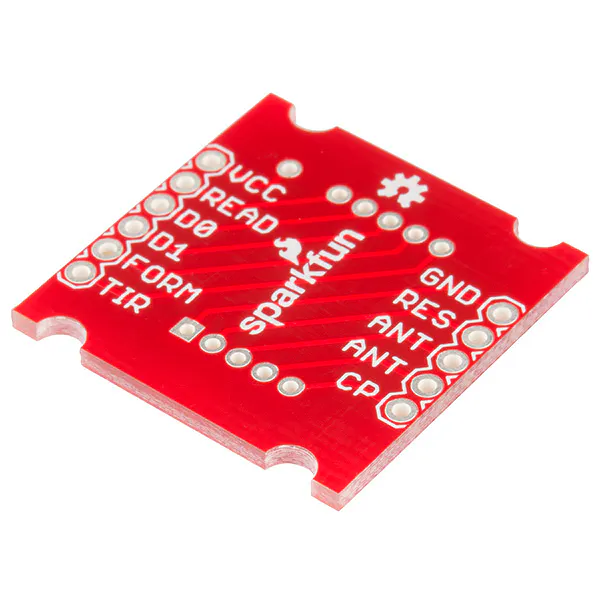SparkFun RFID Reader Breakout
×1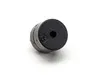Buzzer
×1

### Software apps and online servicesArduino IDE

Read more

## Schematics

### Circuit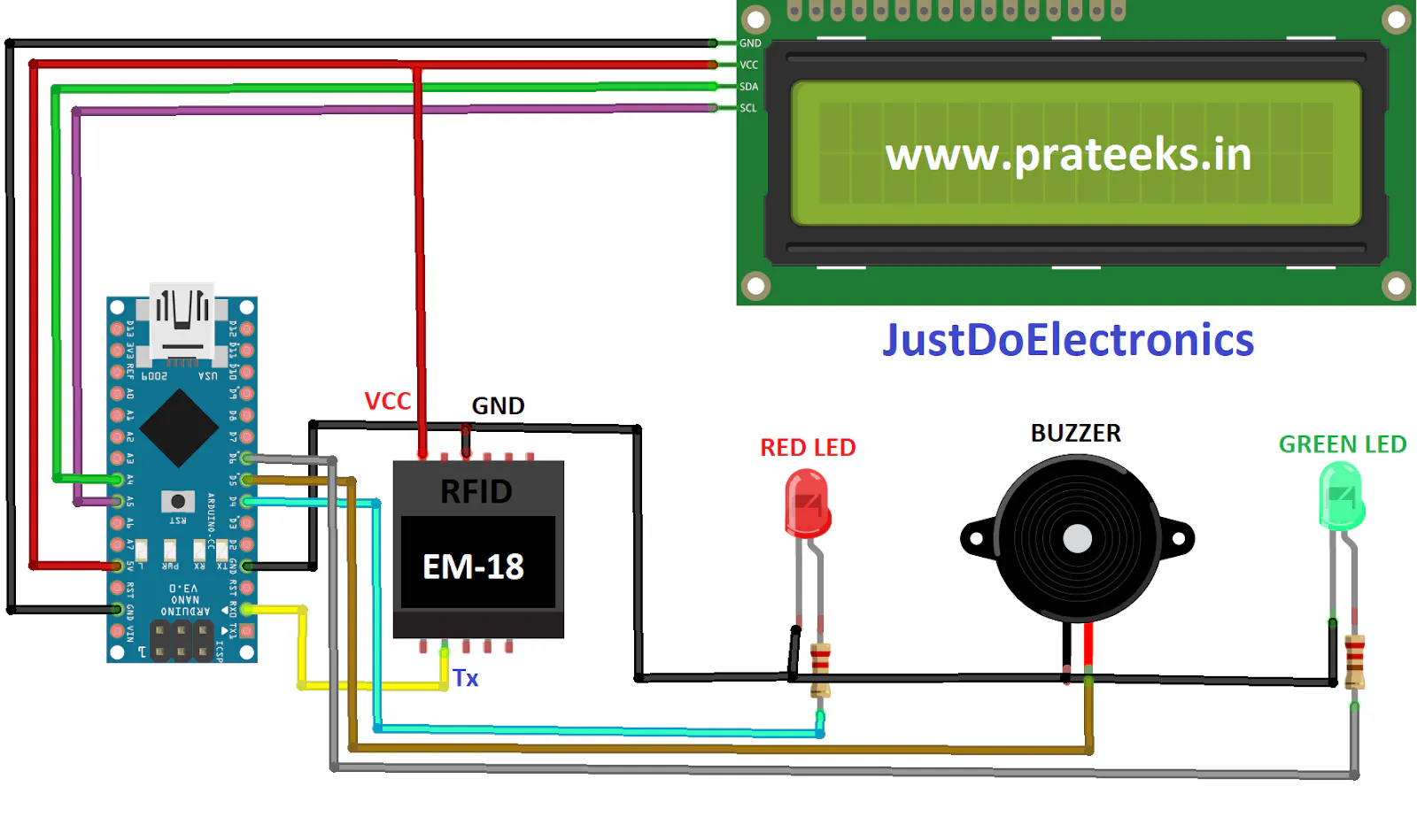## Code

### Code

Arduino
```#include <Arduino.h>

#include <LiquidCrystal_I2C.h>
#include <Wire.h>

LiquidCrystal_I2C lcd(0x27, 16, 2);

char input;
int count = 0;
int a;
int p1=0,p2=0,p3=0,p4=0;
int c1=0,c2=0,c3=0,c4=0;

double total = 0;
int count_prod = 0;

void setup ()
{
//  Pin direction

pinMode(3, INPUT_PULLUP);   //Push Switch
pinMode(4, OUTPUT);         //RED LED
pinMode(5, OUTPUT);         //BUZZER
pinMode(6, OUTPUT);         //GREEN LED

// Initialization
Serial.begin(9600);

//  lcd.begin();
lcd.init();
lcd.backlight();
lcd.setCursor(0, 0);
lcd.print(" AUTOMATIC BILL");
delay (2000);
lcd.setCursor(0, 1);
lcd.print("  SHOPPING CART       ");
delay (2000);
lcd.clear();
lcd.setCursor(0, 0);
lcd.print("   WELCOME TO ");
lcd.setCursor(0, 1);
lcd.print("JUSTDOELECTRONIC");

}
void loop()
{

count = 0;
while (Serial.available() && count < 12)
{
input[count] = Serial.read();
delay(5);
}
int a=digitalRead(3);

if ((strncmp(input, "4000350ABAC5", 12) == 0) && (a == 1))
{

lcd.setCursor(0, 0);
lcd.print("Butter Added       ");
lcd.setCursor(0, 1);
lcd.print("Price(Rm):4.00      ");
digitalWrite(4,HIGH);
digitalWrite(5,LOW);
digitalWrite(6,HIGH);
delay(2000);
total = total + 4.00;
count_prod++;
digitalWrite(4,LOW);
digitalWrite(5,HIGH);
digitalWrite(6,LOW);

}

else if ((strncmp(input, "4000350ABAC5", 12) == 0) && (a == 0))
{
if(p1>0)
{
lcd.clear();
lcd.setCursor(0, 0);
lcd.print("Butter Removed!!!        ");
digitalWrite(4,HIGH);
digitalWrite(5,LOW);
digitalWrite(6,HIGH);
delay(2000);
total = total - 4.00;
lcd.clear();
digitalWrite(4,LOW);
digitalWrite(5,HIGH);
digitalWrite(6,LOW);
}
else
{
lcd.clear();
lcd.setCursor(0, 0);
lcd.print("Not in cart!!!        ");
digitalWrite(4,HIGH);
digitalWrite(5,LOW);
digitalWrite(6,HIGH);
delay(2000);
digitalWrite(4,LOW);
digitalWrite(5,HIGH);
digitalWrite(6,LOW);
lcd.clear();
}
}

if ((strncmp(input, "4000351B7B15", 12) == 0) && (a == 1))
{
lcd.setCursor(0, 0);
lcd.print("Milk Added       ");
lcd.setCursor(0, 1);
lcd.print("Price(Rm):6.00      ");

digitalWrite(6,HIGH);
delay(2000);
total = total + 6.00;
count_prod++;
digitalWrite(4,LOW);
digitalWrite(5,HIGH);
digitalWrite(6,LOW);

}

else if ((strncmp(input, "4000351B7B15", 12) == 0) && (a == 0))
{
if(p2>0)
{
lcd.clear();
lcd.setCursor(0, 0);
lcd.print("Butter Removed!!!        ");
digitalWrite(4,HIGH);
digitalWrite(5,LOW);
digitalWrite(6,HIGH);
delay(2000);

lcd.clear();
digitalWrite(4,LOW);
digitalWrite(5,HIGH);
digitalWrite(6,LOW);
}
else
{
lcd.clear();
lcd.setCursor(0, 0);
lcd.print("Not in cart!!!        ");
digitalWrite(4,HIGH);
digitalWrite(5,LOW);
digitalWrite(6,HIGH);
delay(2000);
digitalWrite(4,LOW);
digitalWrite(5,HIGH);
digitalWrite(6,LOW);
lcd.clear();
}
}

if ((strncmp(input, "4000355181A5", 12) == 0) && (a == 1))
{

lcd.clear();
lcd.setCursor(0, 0);
lcd.print("Total Price :-");

lcd.setCursor(0, 1);
lcd.print(total);

delay(5000);
lcd.clear();
lcd.setCursor(0, 0);
lcd.print("  THANKS FOR  ");
lcd.setCursor(0, 1);
lcd.print("   VISITING ");

}

}
```

## Credits

### Daini

30 projects • 7 followers